﻿ 基于协同过滤算法的移动智能学习平台的开发与设计 Development and Design of Mobile Intelligent Learning Platform on Collaborative Filtering

Software Engineering and Applications
Vol. 08  No. 03 ( 2019 ), Article ID: 30830 , 11 pages
10.12677/SEA.2019.83013

Development and Design of Mobile Intelligent Learning Platform on Collaborative Filtering

Kaixin Yang, Yawei Li

College of Software, Tianjin Normal University, Tianjin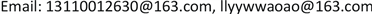Received: May 28th, 2019; accepted: June 12th, 2019; published: June 19th, 2019ABSTRACT

In the education app market, there are fewer comprehensive education apps only providing online course platform learning services. Some education classes only provide the status of teaching assistant functions. We have proposed a teaching aid for colleges and universities. This paper put forward a mobile intelligent learning platform for students to learn online courses. The platform introduces the KNNBaseline collaborative filtering algorithm, proposes to first calculate the similarity between the target user and the user preset by the system, and then perform similarity calculation with the remaining users, effectively solving the problem. The information is too sparse to cause problems, and introduces a baseline model in which the user’s score changes over time. Experiments were carried out using the MovieLens ml-100k dataset. The experimental results show that the algorithm can effectively provide personalized recommendation services for students.

Keywords:Collaborative Filtering, KNNBaseline, Scikit-Surprise, Online Class Learning, Educational Administration, WeChat Applet1. 引言

1.1. 项目开发背景

1.2. 项目开发内容及目的

2. 系统分析与设计

2.1. 系统功能设计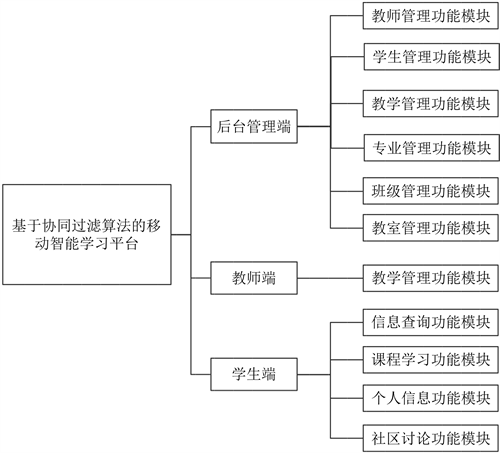Figure 1. The functional module diagram of system 

2.2. 系统架构

1) 后台子系统

2) 课程推荐子系统

3) 用户客户端子系统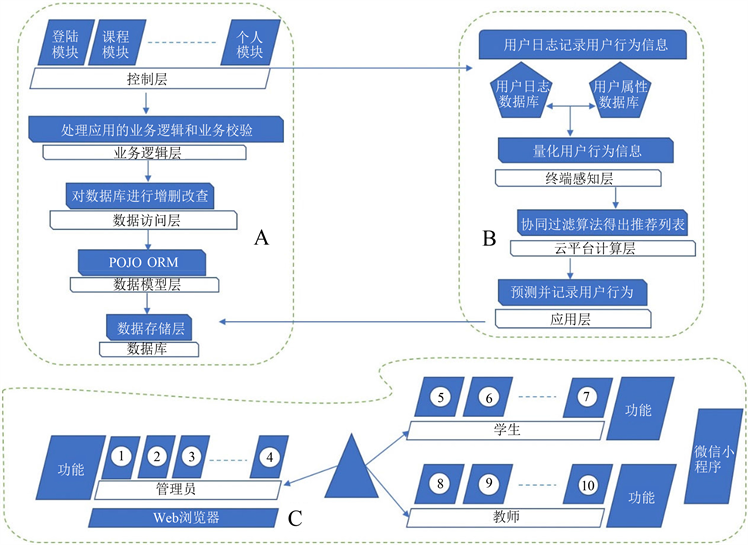Figure 2. System architecture diagram 

2.3. 数据流图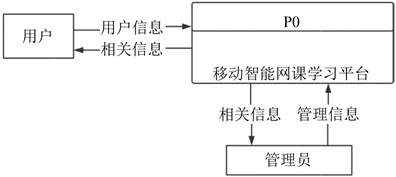Figure 3. Top-level data flow diagram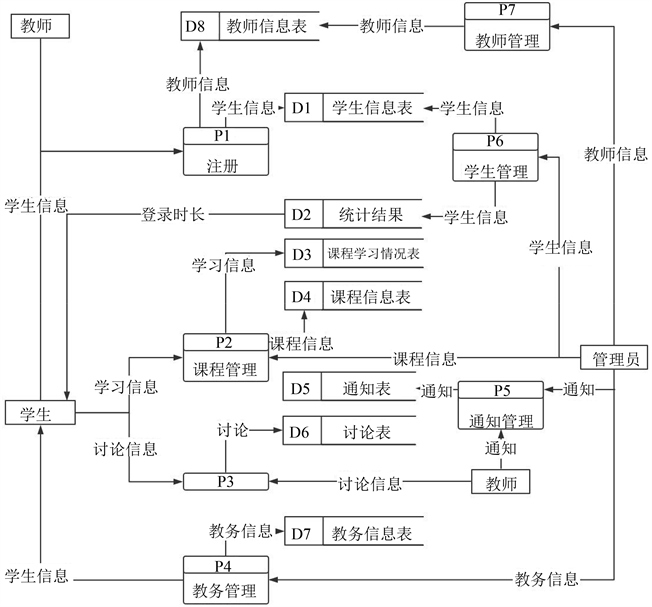Figure 4. First-level data flow diagramFigure 5. Second-level data flow diagram 

3. 系统数据库设计

3.1. 数据库概念设计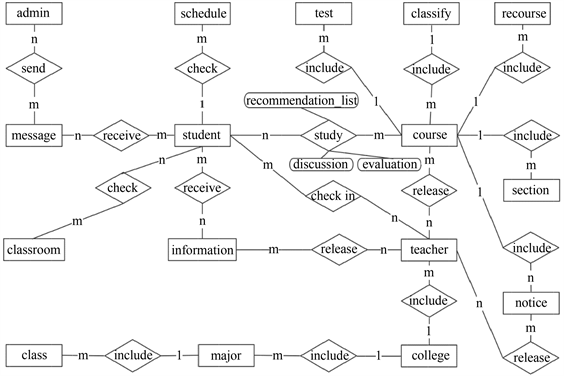Figure 6. E-R diagram of system 

3.2. 数据库逻辑结构设计

4. 系统开发

4.1. 系统开发环境

4.2. 开发技术

4.2.1. 客户端技术方案

4.2.2. 服务器技术方案

5. 基于用户行为信息相似度的协同过滤算法

SciPy是一个开源的基于Python的科学计算工具包。Scikit-surprise，是基于SciPy针对机器学习推荐系统领域发展出的一个简便的推荐系统引擎。本系统使用了协同过滤算法，在传统的协同过滤算法上，引入了一个随时间变化的模型来刻画用户的评分之间的相似度  。传统的协同过滤算法，简单来说是利用用户之间的兴趣相投，共同喜好为用户推荐其感兴趣的信息  ，其基本思想是利用用户对各类课程进行评分获得的矩阵，在用户群中寻找高度相似的用户群，然后综合这些用户对同一信息的评价，预测目标用户对此信息的喜好程度  。协同过滤的实现一般分为两步：首先，获得用户对其所喜好课程的评价信息，再构造评分矩阵，当用户和课程种类的数量都很大时，由于用户通常只对感兴趣的产品进行评分，因此建立的评分矩阵是个巨大的稀疏矩阵Pm * n，见表2Table 2. The information of user's course score table

-代表用户未对此课程进行评分。

${b}_{ui}=\mu +{b}_{u}+{b}_{i}$ (1)

$\mu$ 代表此课程的所有已评分用户的平均得分， ${b}_{u}$ 是用户的偏置， ${b}_{i}$ 是item的偏置。

${b}_{i}=\frac{{\sum }_{u\in R\left(i\right)}\left({r}_{ui}-\mu -{b}_{u}\right)}{\lambda +|R\left(i\right)|}$ (2)

${b}_{u}=\frac{{\sum }_{u\in R\left(u\right)}\left({r}_{ui}-\mu -{b}_{u}\right)}{\lambda +|R\left(u\right)|}$ (3)

6. 课程推荐系统的实现

6.1. 系统开发思想

6.2. 算法步骤

$sim\left(u,v\right)={\rho }_{\stackrel{^}{u}v}=\frac{{\sum }_{i\in {I}_{uv}}\left({r}_{ui}-{b}_{ui}\right)\left({r}_{vi}-{b}_{vi}\right)}{\sqrt{{\sum }_{i\in {I}_{uv}}{\left({r}_{ui}-{b}_{ui}\right)}^{2}}\sqrt{{\sum }_{i\in {I}_{uv}}{\left({r}_{vi}-{b}_{vi}\right)}^{2}}}$ (4)

$sim\left(u,n\right)={\rho }_{\stackrel{^}{m}n}=\frac{{\sum }_{i\in {I}_{uv}}\left({r}_{ui}-{b}_{ui}\right)\left({r}_{ni}-{b}_{ni}\right)}{\sqrt{{\sum }_{i\in {U}_{ij}}{\left({r}_{ui}-{b}_{ui}\right)}^{2}}\sqrt{{\sum }_{i\in {U}_{un}}{\left({r}_{ni}-{b}_{ni}\right)}^{2}}}$ (5)

${r}_{\stackrel{^}{u}i}={b}_{ui}+\frac{{\sum }_{v\in {N}_{i}^{k}\left(u\right)}sim\left(u,v\right)\left({r}_{vi}-{b}_{vi}\right)}{{\sum }_{v\in {N}_{i}^{k}\left(u\right)}sim\left(u,v\right)}$ (6)

${r}_{\stackrel{^}{u}i}={b}_{ui}+\frac{{\sum }_{j\in {N}_{u}^{k}\left(u\right)}sim\left(u,n\right)\left({r}_{ui}-{b}_{ui}\right)}{{\sum }_{j\in {N}_{i}^{k}\left(u\right)}sim\left(u,n\right)}$ (7)

7. 实验设计与结果分析

7.1. 实验准备

7.2. 实验结果分析Figure 7. The result of experiment

7.3. 结果分析

$MAE=\frac{1}{|R^|}{\sum }_{{r}_{\stackrel{^}{u}i}\in R^}|{r}_{ui}-{r}_{\stackrel{^}{u}i}|$ (8)

$RMSE=\sqrt{\frac{1}{|{R}^{\wedge }|}{\sum }_{{r}_{\stackrel{^}{u}i}\in R^}{\left({r}_{ui}-{r}_{\stackrel{^}{u}i}\right)}^{2}}$ (9)

8. 结语

Development and Design of Mobile Intelligent Learning Platform on Collaborative Filtering[J]. 软件工程与应用, 2019, 08(03): 104-114. https://doi.org/10.12677/SEA.2019.83013

1. 1. 极光大数据: 2017年8月在线教育市场研究报告[EB/OL]. http://www.199it.com/archives/637393.html, 2017-09-26.

3. 3. 汪志华, 范祥林, 郁书好. 高校实验室管理系统开发与设计[J]. 软件工程与应用, 2018, 7(4): 218-223.

4. 4. 王渊博, 陈有波. 农产品直卖平台服务端软件设计[J]. 现代电子技术, 2019(8): 171-174.

5. 5. 张洪伟. JSP网络开发技术与整合应用[M]. 北京: 清华大学出版社, 2006.

6. 6. 宋雨欣. 医院预约挂号平台的设计与实现[D]: [硕士学位论文]. 郑州: 郑州大学, 2016.

7. 7. 赵鑫. 医院预约挂号平台的设计与实现论文[EB/OL]. https://wenku.baidu.com/view/0928433ab94ae45c3b3567ec102de2bd9605dec4.html, 2017-09-08.

8. 8. 安忠, 佟志臣. 管理信息系统[M]. 第二版. 北京: 中国铁道出版社, 2006.

9. 9. 刘莹. 基于微信的高校自主学习平台设计与实现[J]. 中国成人教育, 2017(20): 18-21.

10. 10. Koren, Y. (2010) Factor in the Neighbors: Scalable and Accurate Collaborative Filtering. ACM Transactions on Knowledge Discovery from Data, 4, 1-24.

11. 11. 徐林. 基于Spark MLlib协同过滤算法的美食推荐系统研究[J]. 吉林大学学报(信息科学版), 2019, 37(2): 181-185.